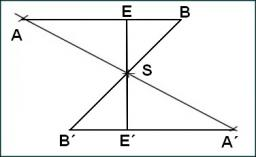# Simple right triangle

Right triangle. Given: page c = 18.8 and angle beta = 22° 23' Calculate page a, b, angle alpha and area.

a =  17.3835
b =  7.1591
α =  67.6167 °
S =  62.225

### Step-by-step explanation:

Try calculation via our triangle calculator.Did you find an error or inaccuracy? Feel free to write us. Thank you!

Tips for related online calculators
Need help calculating sum, simplifying, or multiplying fractions? Try our fraction calculator.
Do you want to convert time units like minutes to seconds?